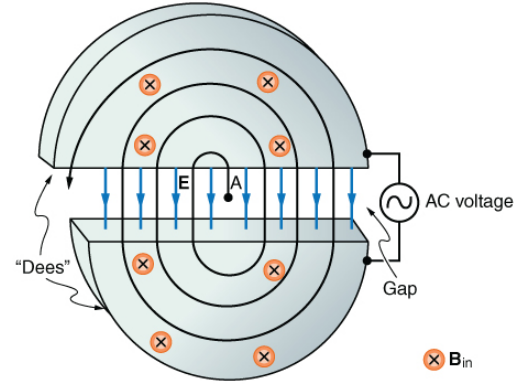# Problem: Integrated Concepts. A cylcotron accelerates charged particles as shown in Figure 22.63.  Using the results of the previous problem, Calculate the frequency of the accelerating voltage needed for a proton in a 1.20-T field. Figure 22.63 Cyclotrons accelerate charged particles orbiting in a magnetic field by placing an AC voltage on the metal Dees, between which the particles move, so that energy is added twice each orbit.  The frequency is constant, since it is independent of the particle energy - the radius of the orbit simply increases with energy until the particles approach the edge and are extracted for various experiments and applications.

###### FREE Expert Solution

Frequency:

$\overline{){\mathbf{f}}{\mathbf{=}}\frac{\mathbf{q}\mathbf{B}}{\mathbf{2}\mathbf{\pi }\mathbf{m}}}$

79% (73 ratings)###### Problem Details

Integrated Concepts. A cylcotron accelerates charged particles as shown in Figure 22.63.  Using the results of the previous problem,
Calculate the frequency of the accelerating voltage needed for a proton in a 1.20-T field.Figure 22.63 Cyclotrons accelerate charged particles orbiting in a magnetic field by placing an AC voltage on the metal Dees, between which the particles move, so that energy is added twice each orbit.  The frequency is constant, since it is independent of the particle energy - the radius of the orbit simply increases with energy until the particles approach the edge and are extracted for various experiments and applications.